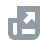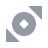Kripto Al
Ödeme yöntemi
Al-Sat
Türevler
Kazan
Finans
NFT
New
Kurumsal
Akış
TRBINANCE.COM
USD

# SSSHesap İşlevleriEğitimiBinance Fan TokenBinance EarnKripto Yatırma/ÇekmeKripto Al (İtibari Para)Spot ve Marjin Alım SatımıKripto Türev İşlemleriFinansAPIGüvenlikKullanım KoşullarıBinance DönüştürmeNFTVIP
Bu makale için şu anda diliniz desteklenmiyor. İngilizce için otomatik çevirmen kullanmanızı öneririz.
Ana sayfa
Destek Merkezi
SSS
Kripto Türev İşlemleri
How to Calculate Liquidation Price of Coin-M Futures Contracts

# How to Calculate Liquidation Price of Coin-M Futures Contracts

2021-02-05 07:59
Below is the liquidation price formula for Coin-M Futures contracts:
where
Notes:
• In Cross Margin mode, WB is crossWalletBalance
• In Isolated Margin mode, WB is Isolated Wallet Balance of the isolated position, where TMM=0 and UPNL=0, You can substitute the position quantity, MMR, cum into the formula to calculate.

## Maintenance Margin Rate

You can find the Maintenance Margin Rate from Leverage and Margin in Coin-Margined Futures Contracts.
For example, if the BTCUSD contract position is 300 BTC, the Maintenance Margin Rate would be 12.5%.
Note: If the position notional (the calculated result of the liquidation price) and the assumed margin levels are different, you must substitute other margin levels’ maintenance margin rate and maintenance margin amount to recalculate the liquidation price.

## Maintenance Amount

You can calculate the Maintenance Amount using the below formula. For example, if the BTCUSD contract position is 300 BTC, the Maintenance Amount would be 11.605 BTC.

### Maintenance Margin Amount formula

Maintenance Margin = Position Notional * Maintenance Margin Rate on the level of position notional - Maintenance Amount on the level of position notional
For example, you have 100 BTCUSDT Perpetual contracts, and the latest Mark Price is 25,000 USDT. The position’s notional value is calculated as 100 * 100 / 25,000 = 0.4 BTC, which is in margin level 1. Thus, you can calculate the corresponding Maintenance Margin Rate and Maintenance Amount and substitute in the above formula:
Maintenance Margin = 100 * 100 / 25,000 * 0.4% - 0 = 0.0016 BTC